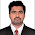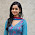## Wednesday, December 2, 2015

### Kullback-Leibler Divergence on Apache Spark

Implementation of the Kullback-Leibler statistical divergence using Scala and Apache Spark resilient distributed datasets.

Overview
The Kullback-Leibler divergence is a concept borrowed from information theory and commonly associated with Information Gain. It is also known as the relative entropy between two distributions. It measures the dissimilarity of the distribution of random values (i.e. probability density function). Let's consider two distribution P and Q with probability density functions p and q
$D_{KL}(P||Q)= - \int_{-\infty }^{+\infty }p(x).log\frac{p(x)}{q(x)}$
The formula can be interpreted as the Information lost when the distribution Q is used to approximate the distribution P
It is obvious that the computation if not symmetric: $D_{KL}(P||Q)\neq D_{KL}(Q||P)$
The Kullback-Leibler divergence is the used to compute the Mutual Information which is one of the feature extraction method. It is part of the family of F-Divergences.

The objective of this post is to implement Kullback-Liebler divergence in Scala, for which the computation is distributed through the Apache Spark framework:
• Implementation of the Kullback-Leibler divergence using Apache Spark
• Illustration of the Kullback-Leibler divergence in comparing multiple continuous probability density functions

Implementation of the Kullback-Liebler Divergence
First let's implement the Kullback-Leibler formula for measuring the dissimilarity between an existing data sets {xi, yi} (presumably, generated by a specific random variable / distribution) and a given continuous distribution with a probability density function f

  1 2 3 4 5 6 7 8 9 10 11 12 13 14 15 16 17 18 19 20 object KullbackLiebler { final val EPS = 1e-10 type DATASET = Iterator[(Double, Double)] def execute( xy: DATASET, f: Double => Double): Double = { val z = xy.filter{ case(x, y) => abs(y) > EPS} - z./:(0.0){ case(s, (x, y)) => { val px = f(x) s + px*log(px/y)} } } def execute( xy: DATASET, fs: Iterable[Double=>Double]): Iterable[Double] = fs.map(execute(xy, _)) } 

The divergence methods execute are defined as static (object methods)
The first invocation of the method execute (line 5) computes the dissimilarity between a distribution that generates the dataset xy with the distribution with a probability density f.
The second execute method computes the KL divergence between a that generates the dataset xy with a sequence of distributions with probability density fs.

Next let's define some of the continuous random distributions used to evaluate the distribution represented by the dataset xy
Normal, beta, lognormal, Gamma, LogGamma and ChiSquare.

  1 2 3 4 5 6 7 8 9 10 11 12 13 14 15 16 17 18 19 20 21 22 23 24 25 26 27 28 29 30 31 32 33 34 35 36 37 38  // One Gaussian distribution val gauss = (x: Double) => INV_PI*exp(-x*x/2.0) // Uniform distribution val uniform = (x: Double) => x // Log normal distribution val logNormal = (x: Double) => { val lx = log(x) INV_PI/x*exp(-lx*lx) } // Gamma distribution val gamma = (x: Double, n: Int) => exp(-x)*pow(x, n)/fact(n) // Log Gamma distribution val logGamma = (x: Double, alpha: Int, beta: Int) => exp(beta*x)*exp(-exp(x)/alpha)/ (pow(alpha, beta)*fact(beta-1)) // Simple computation of m! (for beta) def fact(m: Int): Int = if(m < 2) 1 else m*fact(m-1) // Normalization factor for Beta val cBeta = (n: Int, m: Int) => { val f = if(n < 2) 1 else fact(n-1) val g = if(m < 2) 1 else fact(m-1) f*g/fact(n+m -1).toDouble } // Beta distribution val beta = (x: Double, alpha: Int, beta: Int) => pow(x, alpha-1)*pow(x, beta-1)/cBeta(alpha, beta) // Chi-Square distribution val chiSquare = (x: Double, k: Int) => { val k_2 = k >>1 pow(x, k_2-1)*exp(-0.5*x) /((1 << k_2)*fact(k_2)) } 

Some of these probability density functions are parameterized (i.e. log gamma has an alpha and beta parameters). The probability density function are implemented as Scala partially applied functions with predefined parameter values. For example...

val gamma2 = gamma( _ : Double, 2)
val beta25 = beta(_: Double, 2, 5)
val chiSquare4 = chiSquare(_: Double, 4)


It is important to mention that the implementation of the probability density functions in the code snippet are not optimizer for performance. There are described merely for illustrate the application of the Kullback-Leibler divergence. Please refer to your favorite Statistics handbook to learn more about these probability distributions.

Spark to the rescue
The executing the Kullback-Leibler formula for a large data set with a significant number of random distributions may be a daunting task. A solution is to use Apache Spark framework to parallelize the computation of the divergence. Here are the steps to follow:
• Partition the original data set
• Broadcast the first sequence of probability density functions
• Execute the Kullback-Leibler formula on each partition using mapPartitions
• Collect then aggregate the divergence factor from each partition

 1 2 3 4 5 6 final val NUM_DATA_POINTS = 10000000 val numTasks: Int = 128 val conf = new SparkConf().setAppName("Kullback-Liebler").setMaster(s"local[\$numTasks]") val sc = new SparkContext(conf) sc.setLogLevel("ERROR") 

Once we define the number of input data points (line 1) and the number of concurrent tasks (line 2), the Apache Spark context sc is configured with an instance of SparkConf (line 4).
Next let's implement the broadcasting mechanism for the batch or sequence of probability density functions.

lazy val pdfs = Map[Int, Double => Double](
1 -> uniform,
2 -> logNormal,
3 -> gamma1,
4 -> gamma2,
5 -> gamma4,
6 -> logGamma12,
7 -> logGamma22,
8 -> beta22,
9 -> beta25,
10 -> chiSquare2,
11 -> chiSquare4
)



The broadcast variable pdfs_broadcast alerts the Apache Spark framework that the probability density function pdfs are to be distributed over all the partition of worker (or data) nodes.
Value broadcasting is heavily used in the machine learning library MLlib to dispatch model coefficients (weights) to the data node for the computation of gradient and loss function.

Finally, let's implement the mapPartitions transformation. The method mapPartitions transform an array of values pair {x, y} into an array of size pdfs.size containing the KL divergence value related to each distribution to be evaluated.

 1 2 3 4 5 6 val kl_rdd = master_rdd.mapPartitions((it:DATASET) => { val pdfsList = pdfs_broadcast.value.map( n => pdfs.get(n).get ) execute(it, pdfsList).iterator } ) 

Finally, the divergence values from each partitions is collected then aggregated using the Scala fold operator :/.

 1 2 3 4 5 6 7 8 val kl_master = kl_rdd.collect val divergences = (0 until kl_master.size by pdfs.size) ./:(Array.fill(pdfs.size)(0.0))( (s, n) => { (0 until pdfs.size).foreach(j => s.update(j, kl_master(n+j))) s }).map( _ / kl_master.length) 

Test results
The purpose of the test was to understand the potential of the Kullback-Leibler divergence to evaluate the dissimilarity between probability distribution.
We selected the Gaussian distribution as the benchmark for which other distributions are to be compared. The comparative results are displayed below:

As expected, the Gaussian and Normal distribution are very similar. However, the divergence between Gauss and the highest order of the Gamma and Beta distributions is quite significant. This can be easily confirmed by comparing the graph of their associated probability density functions.

References
Pattern Recognition and Machine Learning - 1.6.1 Relative entropy and mutual information C. Bishop - Springer Science - 2006
Apache Spark documentation

1.Good Tutorials. IF it will be more useful if the same Kullback-Leibler Divergence on Apache Spark in java version. If possible suggest some ideas to implement the same in java or can you provide the same in java since, I am new to Apache Spark

2.These provided information was really so nice,thanks for giving that post and the more skills to develop after refer that post. Your articles really impressed for me,because of all information so nice.

SAP training in Chennai

3.Very interesting tutorials in divergence on apache. Something that students will find very useful to use to integrate, like the tutorials from Essay thinker review which are very helpful and informative.

4.Thanks, but I think the method execute (line 5) has a small bug.

The minus operator on line 10 should be removed.

Otherwise, your code says the formula - ( ∑ p(x) ln p(x) - ∑ p(x) ln q(x) ) = ∑ p(x) ln q(x) - ∑ p(x) ln p(x).
That's not true Kullback–Leibler divergence, i.e the formula must be ∑ p(x) ln p(x) - ∑ p(x) ln q(x).

Maybe you intended to do like below

val z = xy.filter{ case(x, y) => abs(y) > EPS}
- z./:(0.0){ case(s, (x, y)) => {
val px = f(x)
s + px*log(y/px)}
}
}